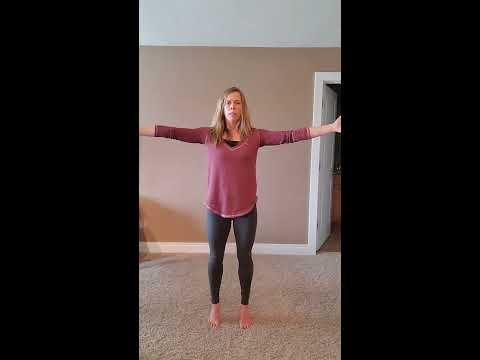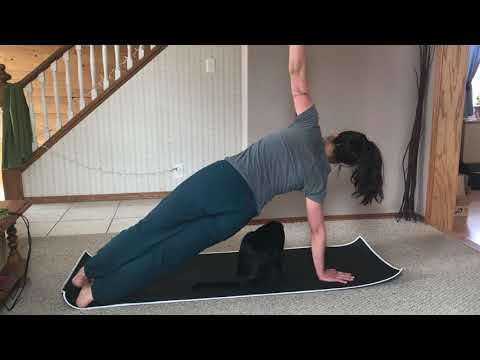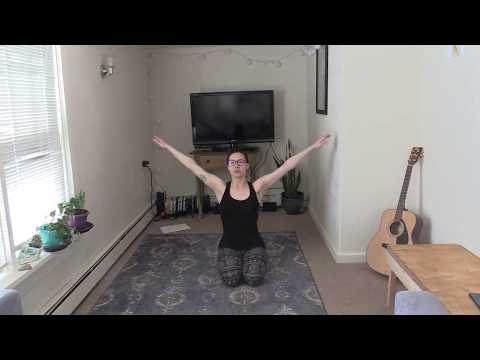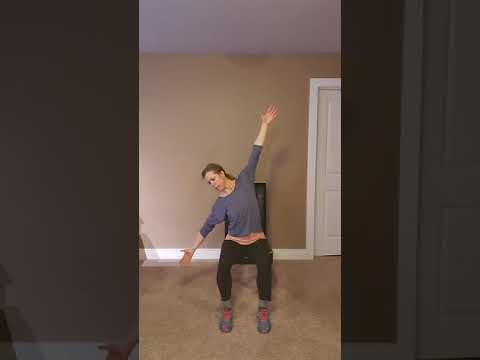# embed test

```/* <![CDATA[ */
\$(function(){
var width = \$(window).innerWidth();
var setwidth = 640;
var ratio = 0.75;
var height = 480;
if(width < setwidth)
{
height = Math.floor(width * 0.75);
//console.log("device width "+width+", set width "+640+", ratio "+0.75+", new height "+ height);
var new_url = wpvl_paramReplace('width', link, width);
var new_url = wpvl_paramReplace('height', new_url, height);
\$("a#6006c665df264").attr('href', new_url);
//console.log(new_url);
}
});
});
/* ]]&gt; */
```
```/* <![CDATA[ */
\$(function(){
var width = \$(window).innerWidth();
var setwidth = 640;
var ratio = 0.75;
var height = 480;
if(width < setwidth)
{
height = Math.floor(width * 0.75);
//console.log("device width "+width+", set width "+640+", ratio "+0.75+", new height "+ height);
var new_url = wpvl_paramReplace('width', link, width);
var new_url = wpvl_paramReplace('height', new_url, height);
\$("a#6006c665df29b").attr('href', new_url);
//console.log(new_url);
}
});
});
/* ]]&gt; */
```

## Lower Body Combination

```/* <![CDATA[ */
\$(function(){
var width = \$(window).innerWidth();
var setwidth = 100%;
var ratio = 1;
var height = 100%;
if(width < setwidth)
{
height = Math.floor(width * 1);
//console.log("device width "+width+", set width "+100%+", ratio "+1+", new height "+ height);
var new_url = wpvl_paramReplace('width', link, width);
var new_url = wpvl_paramReplace('height', new_url, height);
\$("a#6006c665df2cb").attr('href', new_url);
//console.log(new_url);
}
});
});
/* ]]&gt; */
```

```/* <![CDATA[ */
\$(function(){
var width = \$(window).innerWidth();
var setwidth = 640;
var ratio = 0.75;
var height = 480;
if(width < setwidth)
{
height = Math.floor(width * 0.75);
//console.log("device width "+width+", set width "+640+", ratio "+0.75+", new height "+ height);
var new_url = wpvl_paramReplace('width', link, width);
var new_url = wpvl_paramReplace('height', new_url, height);
\$("a#6006c665df2fc").attr('href', new_url);
//console.log(new_url);
}
});
});
/* ]]&gt; */
```
```/* <![CDATA[ */
\$(function(){
var width = \$(window).innerWidth();
var setwidth = 640;
var ratio = 0.75;
var height = 480;
if(width < setwidth)
{
height = Math.floor(width * 0.75);
//console.log("device width "+width+", set width "+640+", ratio "+0.75+", new height "+ height);
var new_url = wpvl_paramReplace('width', link, width);
var new_url = wpvl_paramReplace('height', new_url, height);
\$("a#6006c665df327").attr('href', new_url);
//console.log(new_url);
}
});
});
/* ]]&gt; */
```
```/* <![CDATA[ */
\$(function(){
var width = \$(window).innerWidth();
var setwidth = 640;
var ratio = 0.75;
var height = 480;
if(width < setwidth)
{
height = Math.floor(width * 0.75);
//console.log("device width "+width+", set width "+640+", ratio "+0.75+", new height "+ height);
var new_url = wpvl_paramReplace('width', link, width);
var new_url = wpvl_paramReplace('height', new_url, height);
\$("a#6006c665df34f").attr('href', new_url);
//console.log(new_url);
}
});
});
/* ]]&gt; */
```
```/* <![CDATA[ */
\$(function(){
var width = \$(window).innerWidth();
var setwidth = 640;
var ratio = 0.75;
var height = 480;
if(width < setwidth)
{
height = Math.floor(width * 0.75);
//console.log("device width "+width+", set width "+640+", ratio "+0.75+", new height "+ height);
var new_url = wpvl_paramReplace('width', link, width);
var new_url = wpvl_paramReplace('height', new_url, height);
\$("a#6006c665df375").attr('href', new_url);
//console.log(new_url);
}
});
});
/* ]]&gt; */
```
```/* <![CDATA[ */
\$(function(){
var width = \$(window).innerWidth();
var setwidth = 640;
var ratio = 0.75;
var height = 480;
if(width < setwidth)
{
height = Math.floor(width * 0.75);
//console.log("device width "+width+", set width "+640+", ratio "+0.75+", new height "+ height);
var new_url = wpvl_paramReplace('width', link, width);
var new_url = wpvl_paramReplace('height', new_url, height);
\$("a#6006c665df394").attr('href', new_url);
//console.log(new_url);
}
});
});
/* ]]&gt; */
```
```/* <![CDATA[ */
\$(function(){
var width = \$(window).innerWidth();
var setwidth = 640;
var ratio = 0.75;
var height = 480;
if(width < setwidth)
{
height = Math.floor(width * 0.75);
//console.log("device width "+width+", set width "+640+", ratio "+0.75+", new height "+ height);
var new_url = wpvl_paramReplace('width', link, width);
var new_url = wpvl_paramReplace('height', new_url, height);
\$("a#6006c665df3b1").attr('href', new_url);
//console.log(new_url);
}
});
});
/* ]]&gt; */
```
```/* <![CDATA[ */
\$(function(){
var width = \$(window).innerWidth();
var setwidth = 640;
var ratio = 0.75;
var height = 480;